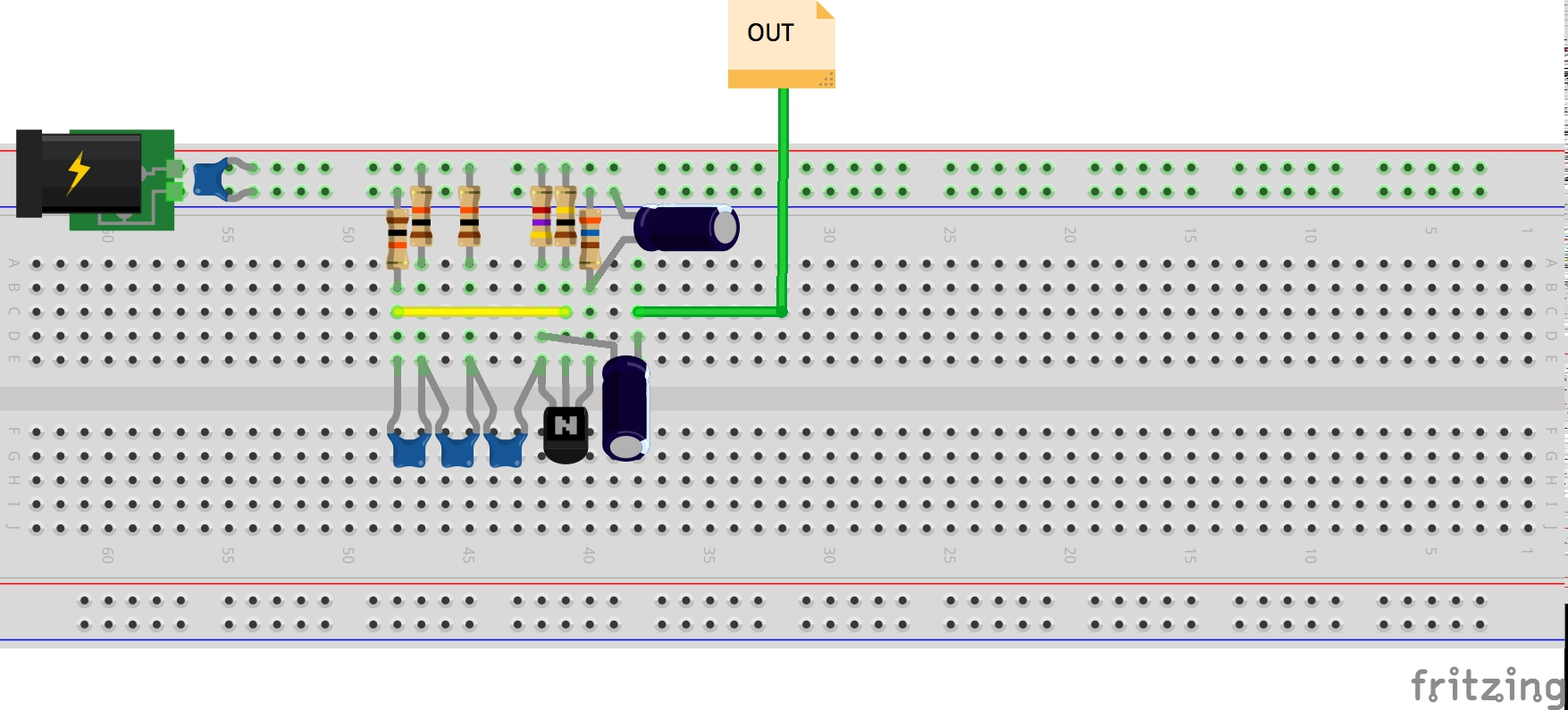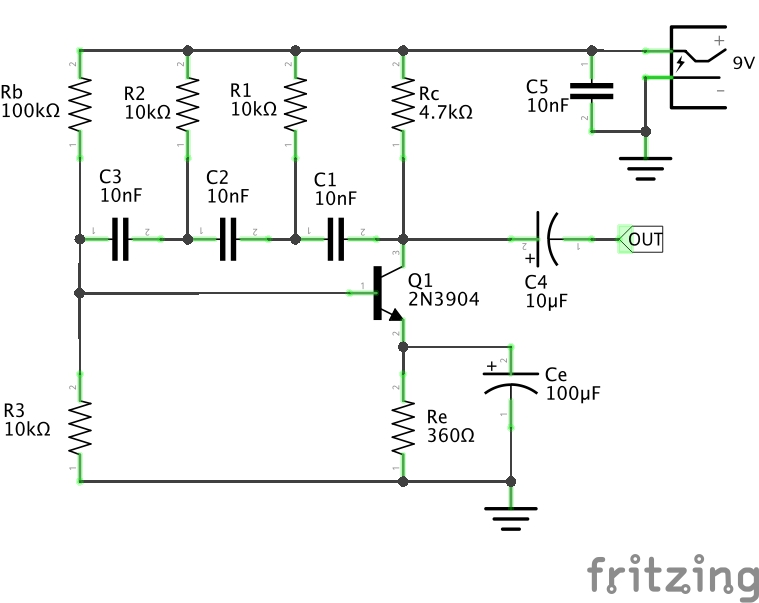#332 Oscillators/RCPhaseShiftBJT

Testing an RC phase-shift oscillator using a BJT amplifier.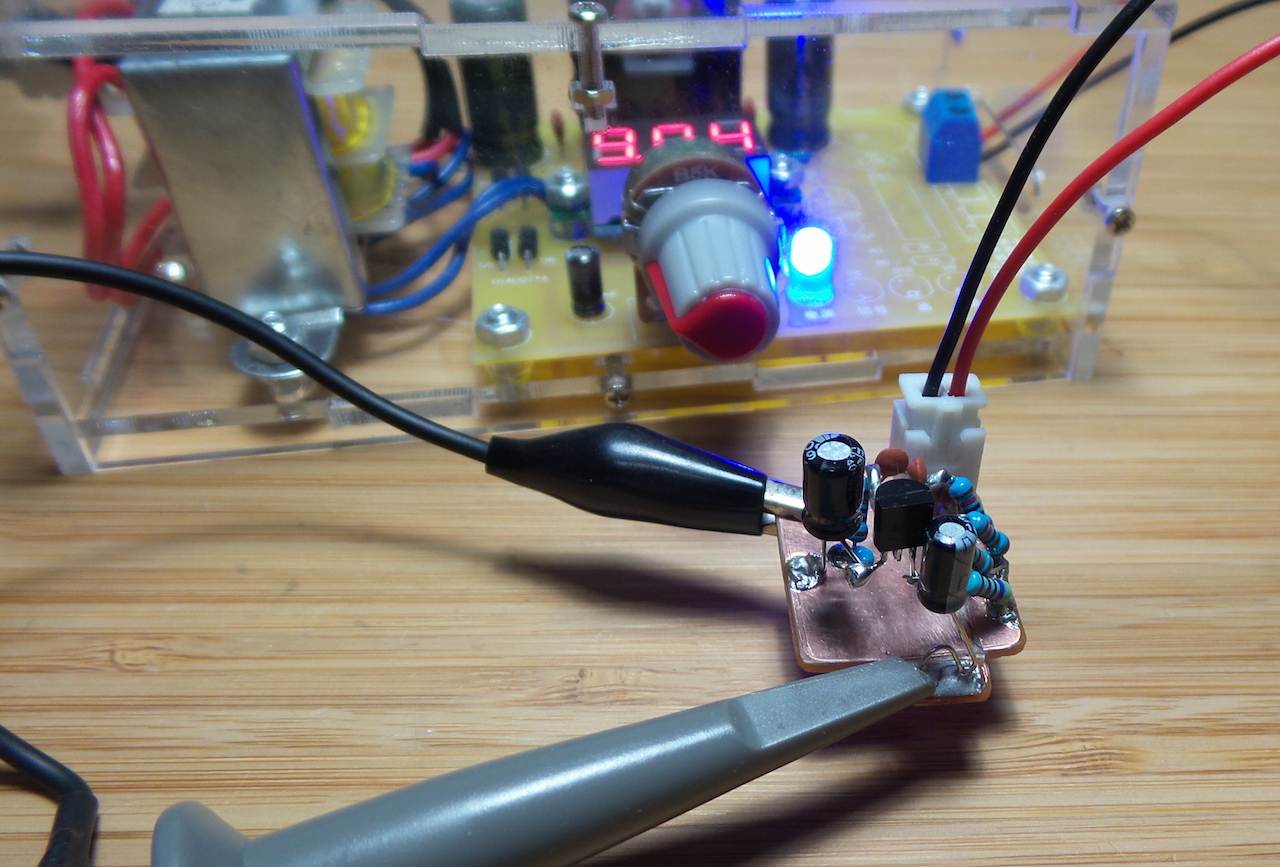Given an inverting amplifier (180˚ phase shift), with output fed back into a network that adds another 180˚ phase shift, and provided the amplifier gain is sufficient to provide overall positive feedback gain of 1 … then we have the basis of an oscillotor.

The RC phase-shift oscillator with BJT amplifier is one of the simplest realisations of this, requiring no special components:

• an NPN BJT in a common-emitter configuration is the inverting amplifier
• a cascade of RC high-pass filters provide the additional 180˚ phase shift
• the amplifier provides enough gain to overcome losses in the RC network

The basic BJT/RC phase shift oscillator is good for low(er) frequency sine wave generation. Main drawbacks:

• performance is very component-specific. Actual frequency may be far of theoretical.
• variable frequency control is non-trivial enhancement

RC High-pass Filter Phase Shift• Capacitive Reactance: `Xc = 1/2πfC`
• Impedance: `Z = √(R² + Xc²)`
• phase angle: `ø = arctan(Xc/R)`

A single filter output leads by at most approaching 90˚, therefore three filters in series are required to achieve ≥ 180˚. More stages could be used, but this adds more error and variation.

The frequency of the filter network is predicted based on the -3dB cut-off: `f = 1/(2πRC√(2N))`, where N is the number of filter stages.

The total phase shift is then: `ø = N.arctan(1/(2πfRC))`

So in the basic R=10kΩ, C=10nF configuration I’m using here, we’d expect frequency of 650Hz with a phase shift for each stage of 67˚, for a total phase shift of 201˚ - safely over the 180˚ minimum required.

Biasing the BJT

The circuit I’m using is probably the canonical demonstration. The BJT is in a Class A common-emitter amplifier configuration with:

• voltage divider bias
• emitter resistor Re. Higher Re stabilises emitter current, making it more independent of the transisitor ß/hFE.
• a bypass capacitor in parallel with Re improves AC gain. Set Xc≤0.10RE for lowest frequency.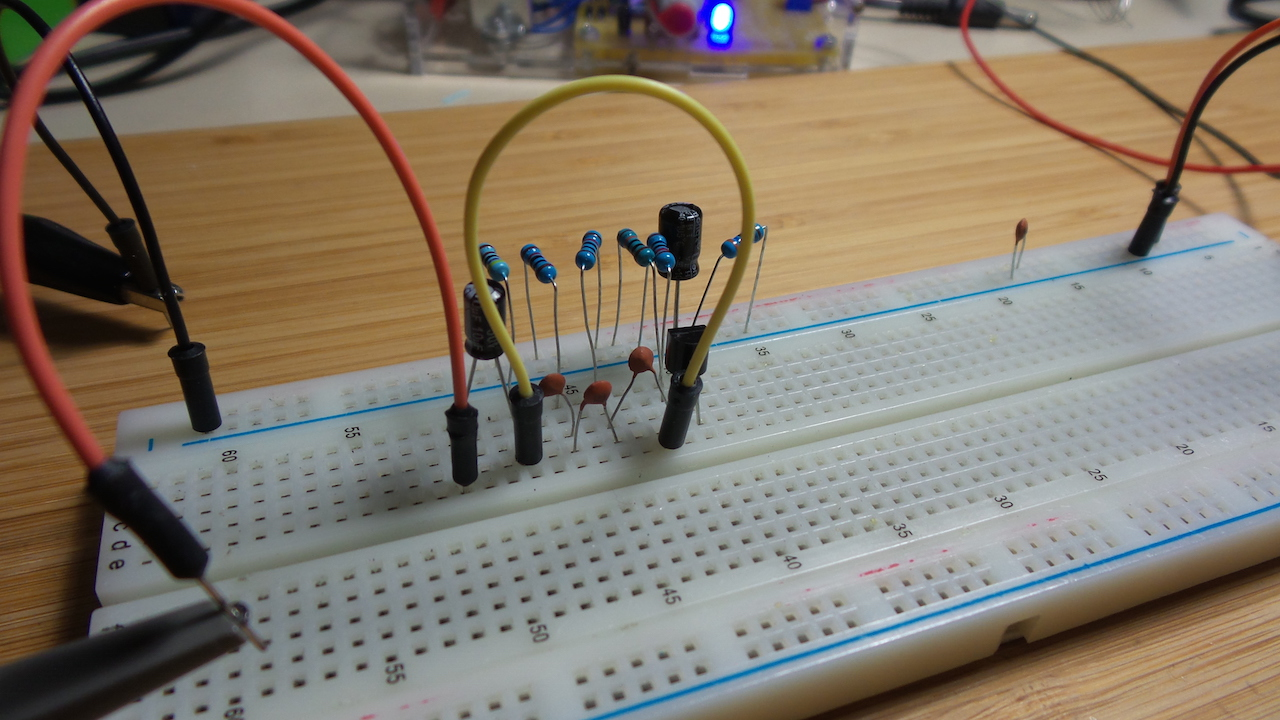Notes and modifications I’ve made after the initial schematic design:

• add C5 (10nF) to smooth the power supply; this helped stabilise the oscillator significantly
• had to play around with the value of Re to ensure the transistor stayed in the linear region. Settled on 360Ω.
• for this biasing arrangement, requires a minimum of 8.5V supply for the oscillator to get started

R=10kΩ, C=10nF

Produced a 746 Hz oscillation in practice, 14% above predicted. Not very surprised - especially on a breadboard. The phase shift per pole of the filter should be around 64˚

With a 9V supply, it produces a very nice sine wave at 3.66V peak-to-peak.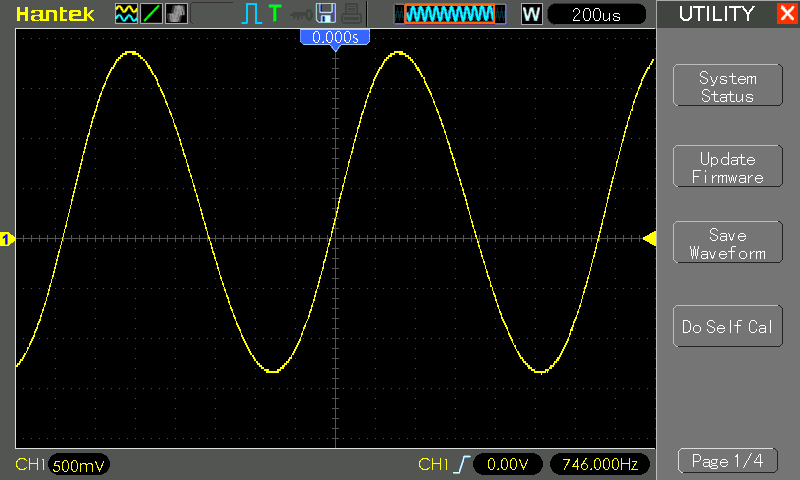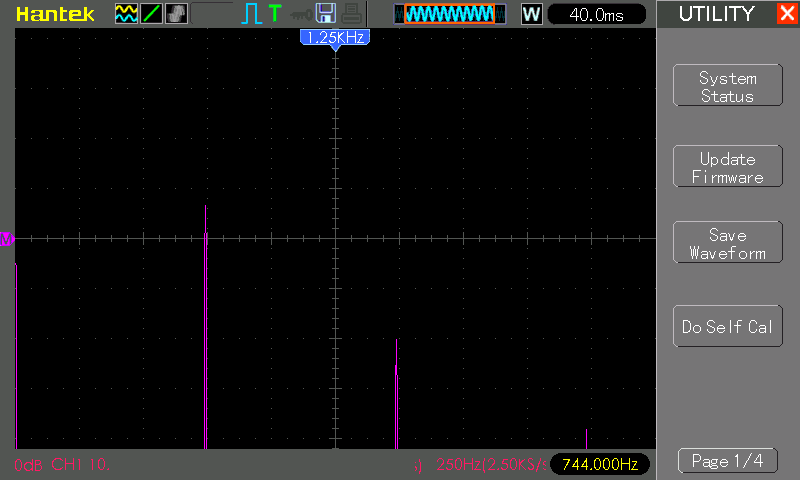R=10kΩ, C=100nF

I randomly switch the capacitors to 100nF and it slows things down to a very nice sine wave at 103Hz.

But this is curious: the phase shift per pole would presumably be 57˚, and one would think the oscillation couldn’t be maintained.

Since I know these ceramic 100nF caps tend to err significantly low (sometimes down to ~90nF), I suspect this is only working for me accidentally doe to parts tolerance.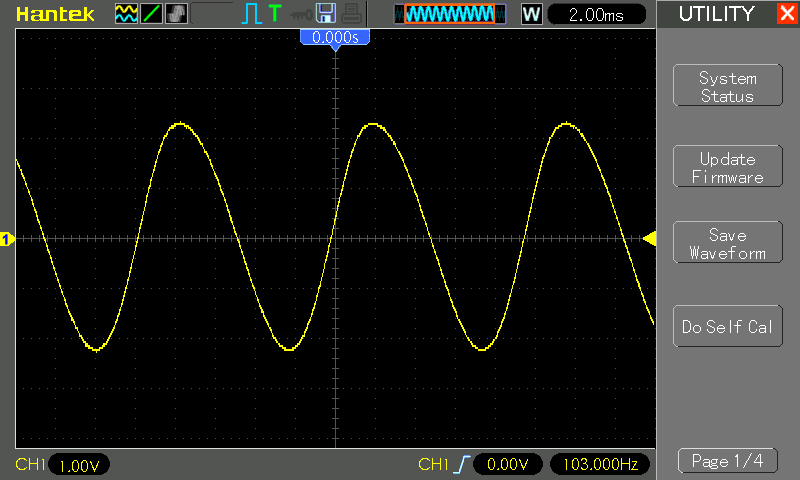Results on a Copper PCB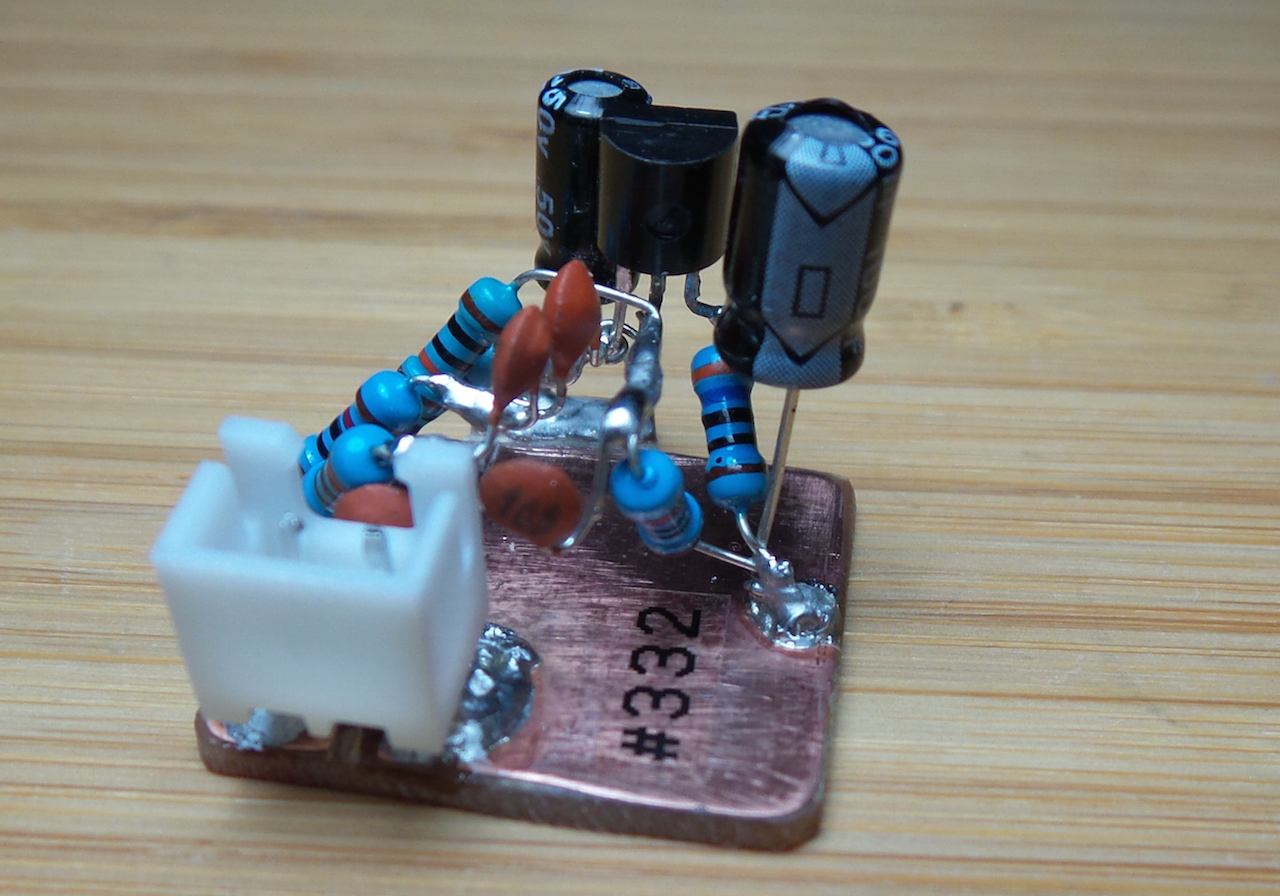Results with an ugly-style construction on a copper PCB are much closer to theory:

• measured frequency is 690Hz - just 6% above theoretical frequency
• at 9V VCC, output sine wave is a strong 4.2V peak-to-peak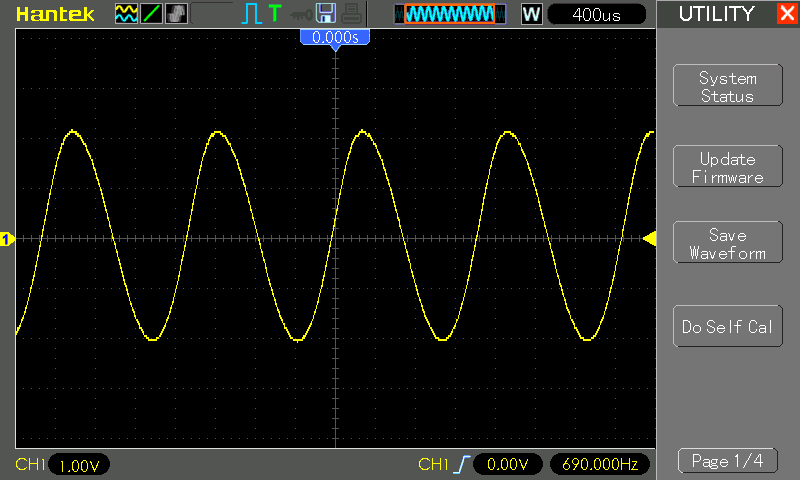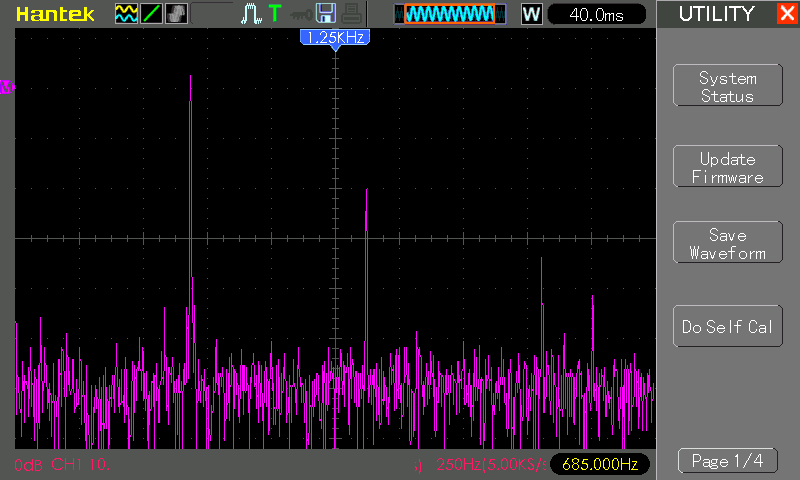Construction